I created a Arduino controled led clock with 60 rgb led’s. This one is inspired by the Equinox Clock from Bram Knaapen.

##### LED strip#

For the 60 RGB leds I use a string of RGB leds that I order directly from China, each of those leds has a LPD6803 IC. A arduino library for this string can be found at adafruit’s github.

##### Time Keeping#

For time keeping I am using a a RTC DS1307 that is connected via I2C to pin A4 and A5 on the Arduino. Pin 7 from the DS1307 is connected to pin D2 on the Arduino an is set to a 1Hz Square Wave. I am using this to give the Arduino every second a interrupt, this made the timing a lot easier. I build the DS1307 on a custom should with breakout pins.

##### Dimming#

There is also a LDR connected at pin A2, this wil measure the light and dim the led’s accordingly.

##### Arduino Shield#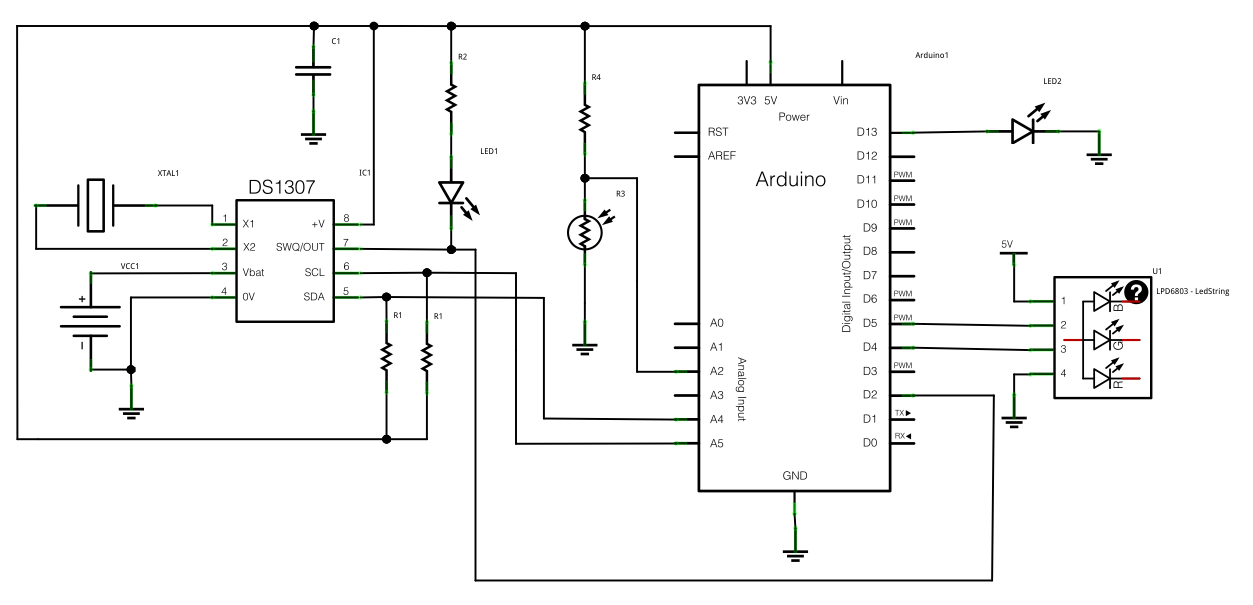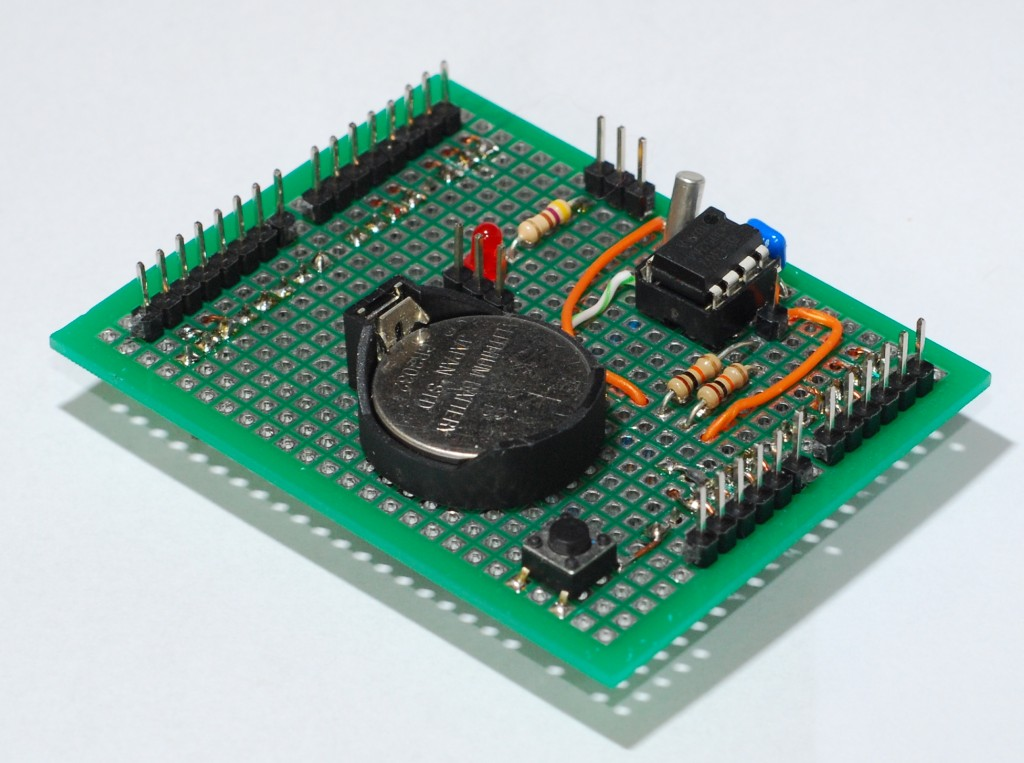##### Design#

For the design of the casing I am going for a 12 angle shape. The edge will be layered with sanded plexiglas sheets for a nice glow effect. The LED’s will in a aluminum bracket. Here is the first design what I made in google sketchup.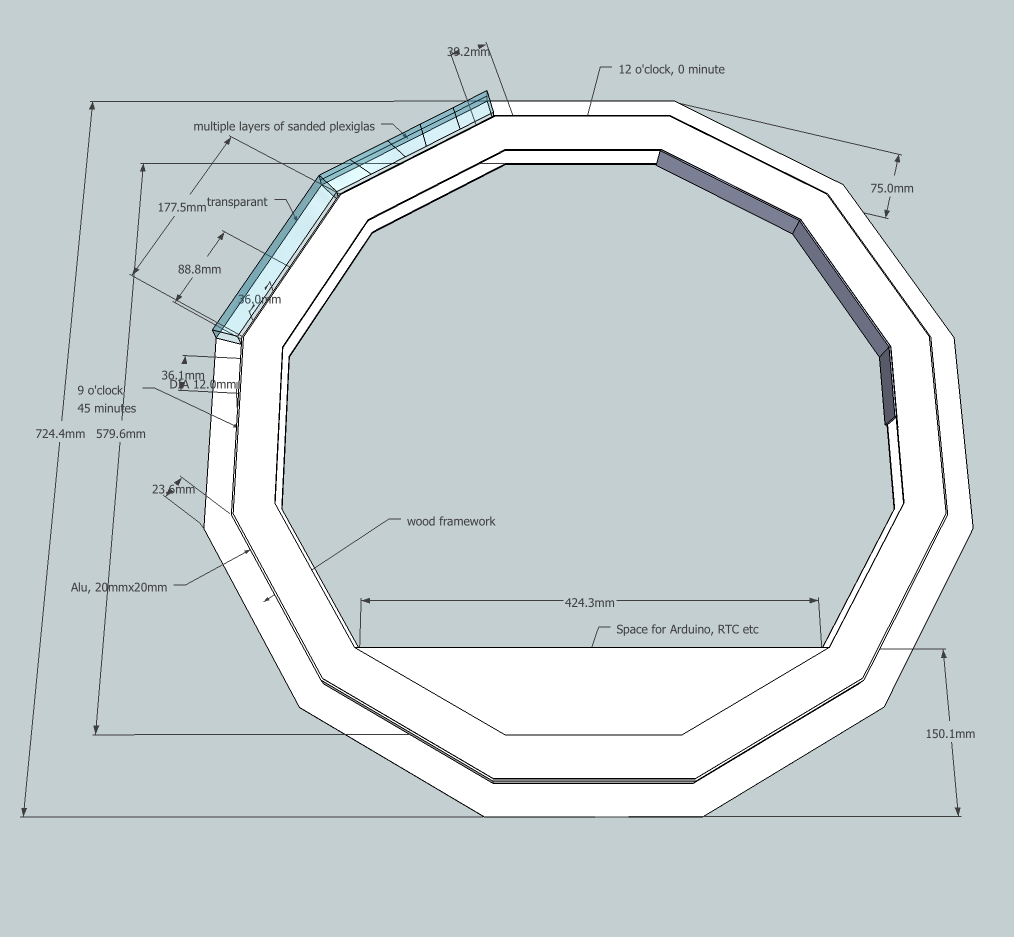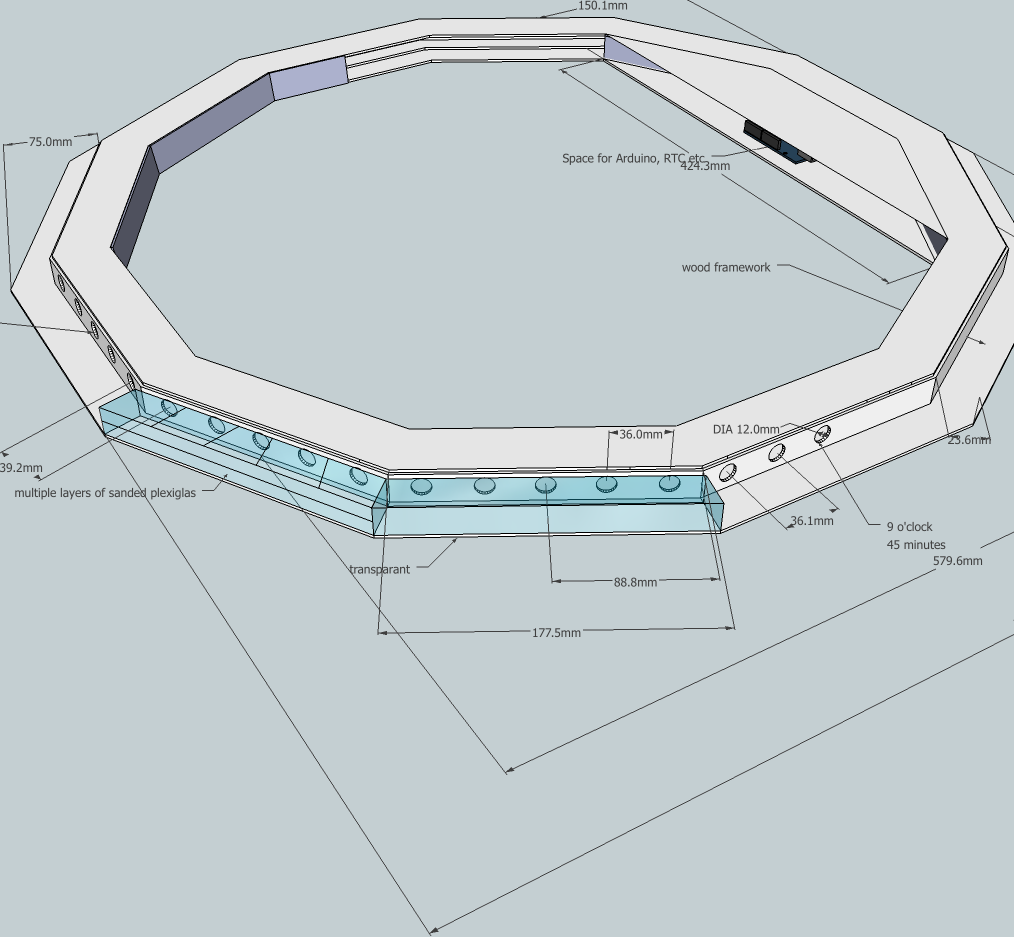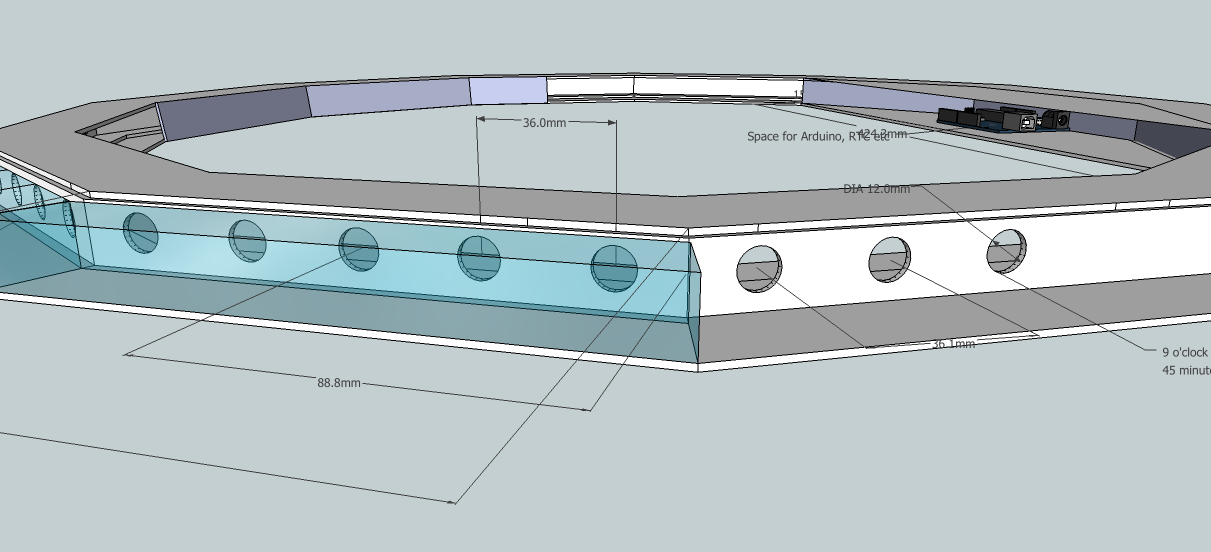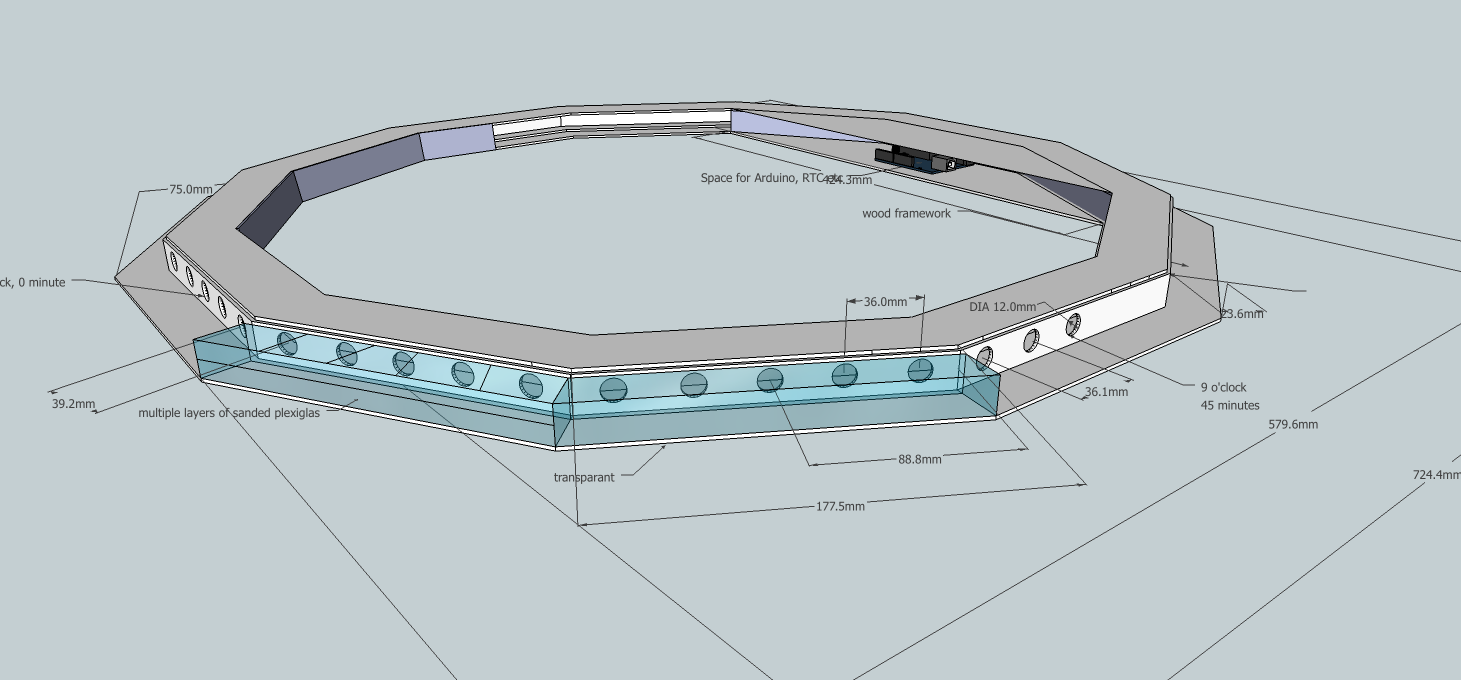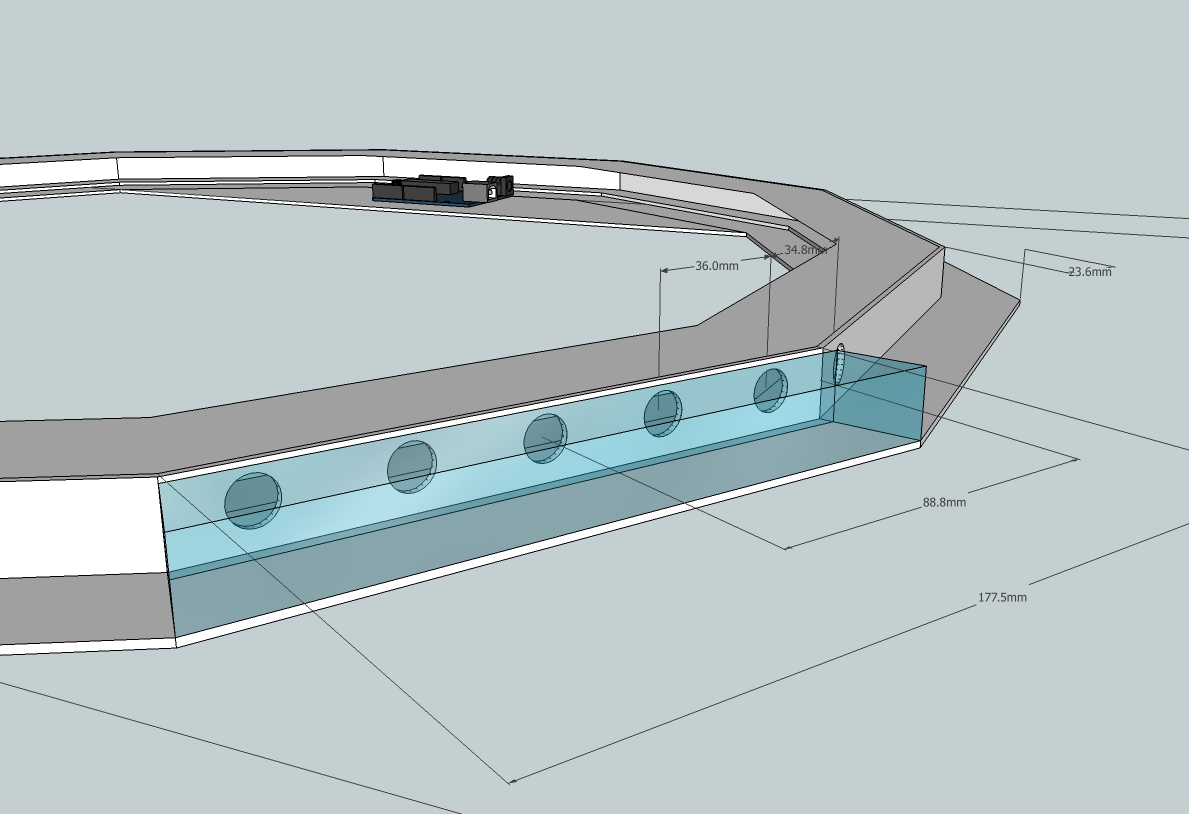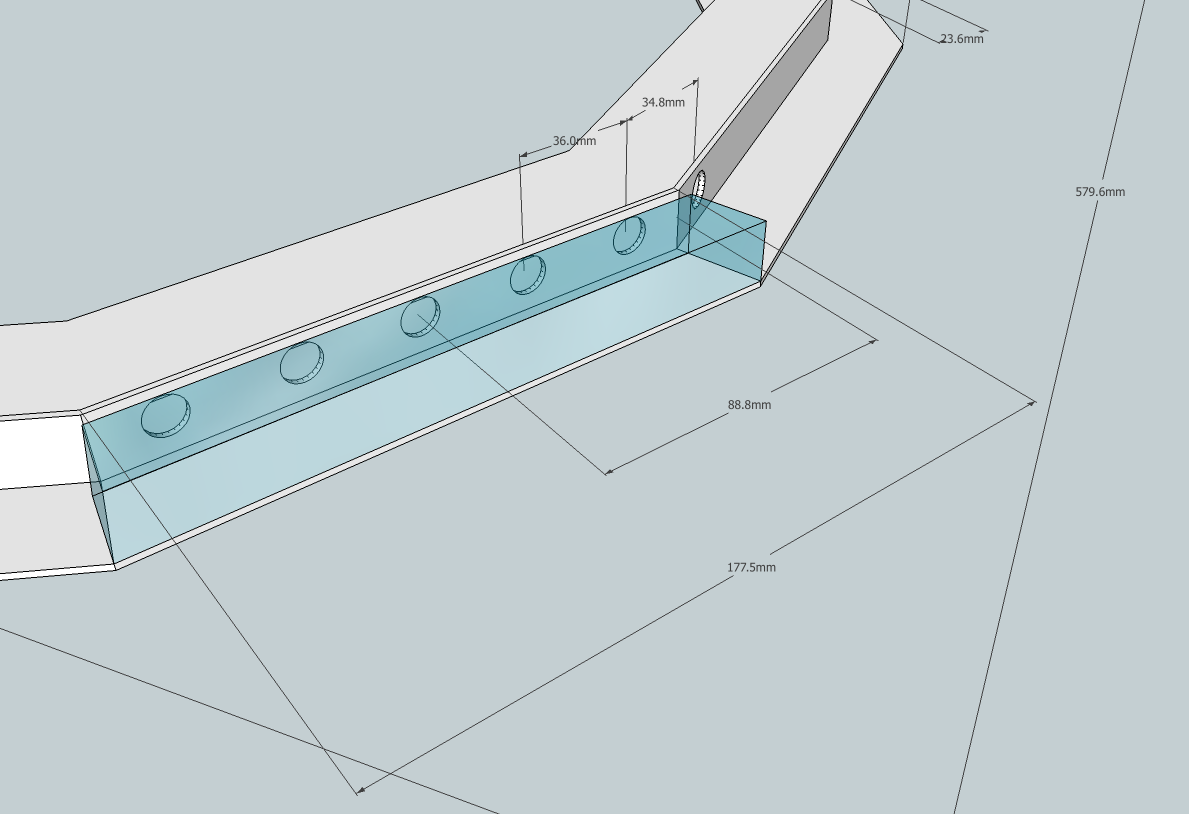##### Power#

The led string if all rgb leds are on will draw about 3.6 Amps at 5 volts. This of course is way to much for the Arduino regulator. But most of the time not all led’s will be on or at full brightness. Now I am using a switched 5.1 volt / 2 Amp power-supply that I had lying around. This power supply is directly connected to the + 5v on the arduino. So it’s bypassing the regulator on the Arduino.

##### Video#

Here is a short video overview from the first proto type, this on is fitted in a carton box, so nothing fancy yet. Now I need to get my hands on a laser cutter to build the casing.

##### Code#

All the files, codes and sources of this project you can find on my github.

``````//
// Equilux, a RGB Led Clock by Dennis Kruyt ([email protected]) 2011
// Inspired by the Equinox Clock from Bram Knaapen.
//
/*****************************************************************************/

#include "LPD6803.h"

// LPD6802 pins
int dataPin = 4;       // 'green' wire
int clockPin = 5;      // 'blue' wire
// Don't forget to connect 'yellow' to ground and 'red' to +5V

// LDR pin
int LDRPin = A2;

// Timer 1 is also used by the strip to send pixel clocks
#include <TimerOne.h>

// Set the first variable to the NUMBER of pixels. 60 = 60 pixels in a row
LPD6803 strip = LPD6803(60, dataPin, clockPin);

//wire and rtc
#include <Wire.h>
#include "RTClib.h"

// RTC_Millis is for a soft rtc
//RTC_Millis RTC;
RTC_DS1307 RTC;

// Define second, minute, hour
int s;
int m;
int h;

int z;

// Set brightness min/max 31
int MinBright = 6;
int MaxBright = 31;
int brightness;

// LDR
int LDRValue;

// Pin 13 is used for a sec blink led
int led = 13;
volatile int state = LOW;

void setup() {

Serial.begin(19200);

//start wire and rtc
Wire.begin();
RTC.begin();

if (! RTC.isrunning()) {
Serial.println("RTC is NOT running!");
// following line sets the RTC to the date & time this sketch was compiled
}

// set 1hz sqw on DS1307 pin 7, we will using this for a interrupt on arduino pin 2
Wire.beginTransmission(0x68);              // write the control register
Wire.send(0x90);                           // 0x90=1Hz, 0x91=4kHz, 0x92=8kHz, 0x93=32kHz
Wire.endTransmission();

// The Arduino needs to clock out the data to the pixels
// this happens in interrupt timer 1, we can change how often
// to call the interrupt. setting CPUmax to 100 will take nearly all all the
// time to do the pixel updates and a nicer/faster display,
// especially with strands of over 100 dots.
// (Note that the max is 'pessimistic', its probably 10% or 20% less in reality)
strip.setCPUmax(95);  // up this if the strand flickers or is slow

// Start up the LED counter
strip.begin();

// Update the strip, to start they are all 'off'
strip.show();

//Attach pin 7 from DS1307 to Arduino pin 2 and call function clock
attachInterrupt(0, clock, FALLING);

// Set pin 13 (led) to output mode
pinMode(led, OUTPUT);

}

// Empty loop, all is done by the 1hz interupt on pin 2
void loop () { }

void clock() {

//attach Interrupt stops the strip, so start it again
strip.begin();

digitalWrite(led, state);
state = !state;

//Get current time
DateTime now = RTC.now();

// mapping hour 24 => 12 => 60
h = now.hour(), DEC;
if (h > 12) { h = h - 12; }
//else if (h = 12) { h == 0; }
h = map(h, 0, 12, 0, 60);

if ( m < 15 ) { h == h; }
else if ( m < 30 ) { h = h + 1; }
else if ( m < 45 ) { h = h + 2; }
else if ( m < 59 ) { h = h + 3; }

m = now.minute(), DEC;
s = now.second(), DEC;

// Get LDR vaulue and set brightness
brightness = map(LDRValue, 0, 1023, MaxBright, MinBright);

// set increase step
if ( brightness < 20 ) {
z = 1;
} else {
z = 2;
}

//todo
//strip.setPixelColor((h - 1), 0, 0, 0);

strip.setPixelColor((m - 1), 0, 0, 0);
//unset -2 ,-3 seconds
strip.setPixelColor((s - 2 ), 0, 0, 0);
strip.setPixelColor((s - 3 ), 0, 0, 0);

// clear transistion from 59 -> 0
if (s == 0) { strip.setPixelColor((58), 0, 0, 0); }
if (s == 1) { strip.setPixelColor((59), 0, 0, 0); }
if (m == 0) { strip.setPixelColor((59), 0, 0, 0); }

for (int y = 1; y < brightness; y = y + z) {

strip.setPixelColor((s - 1 ), (brightness - y), 0, 0);
if (s == 0) { strip.setPixelColor(59, (brightness - y), 0, 0); }
strip.setPixelColor(s, brightness, 0, 0);
strip.setPixelColor((s + 1 ), y, 0, 0);
if (s == 59) { strip.setPixelColor(0, y, 0, 0); }

strip.setPixelColor(m, 0, brightness, 0);
strip.setPixelColor(h, 0, 0, brightness);

// second equals minute
if ((s + 1) == m) { strip.setPixelColor(s + 1, y, brightness, 0); }
if (s == m) { strip.setPixelColor(s, brightness, brightness, 0); }
if ((s - 1) == m) { strip.setPixelColor(s - 1, (brightness - y), brightness, 0); }

// second equals hour
if ((s + 1) == h) { strip.setPixelColor(s + 1, y, 0, brightness); }
if (s == h) { strip.setPixelColor(s, brightness, 0, brightness); }
if ((s - 1) == h) { strip.setPixelColor(s - 1, (brightness - y), 0, brightness); }

// update strip
strip.show();

delay(105 - (2 * y));
}

// Debug
//Serial.print(now.year(), DEC);
//Serial.print('/');
//Serial.print(now.month(), DEC);
//Serial.print('/');
//Serial.print(now.day(), DEC);
//Serial.print(' ');
//Serial.print(now.hour(), DEC);
//Serial.print(':');
//Serial.print(now.minute(), DEC);
//Serial.print(':');
//Serial.print(now.second(), DEC);
//Serial.println();
//Serial.println(h);

}
``````